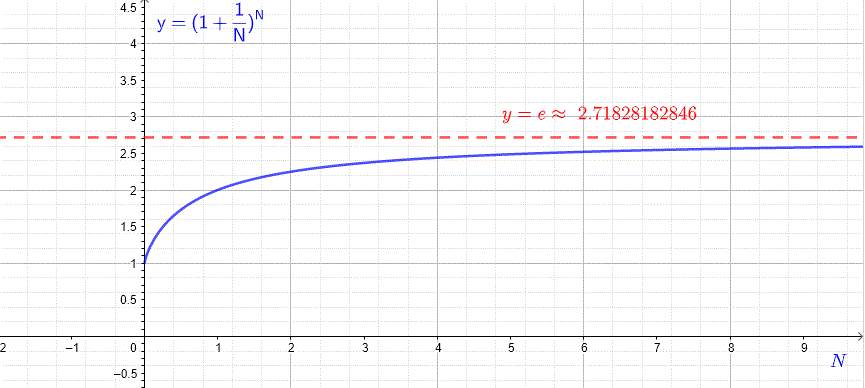# Euler Constant e

## Euler Constant e

In compounding of interest it was shown that if, for example, an amount of money P (principal) is invested at an annual percentage rate r, the total amount of money A after t years is given by
$A = P(1 + r)^t$
It was also shown that if the interest is compounded n times during each year, the amount of money after t years is given by
$A = P(1 + r/n)^{nt}$
Let $N = n / r$ , then $r / n = 1 / N$ and $n = r N$ , hence the formula for A becomes
$A = P(1 + 1 / N)^{N r t}$
Which can be written as
$A = P ( (1 + 1 / N)^N )^{r t}$
The question that one may ask is that what if we increase n indefinitely?
As the number of compounding n increases, $N$ also increases, the term $(1 + 1 / N)^N$ approaches a constant value called $e$ (after the swiss mathematician Leonhard Euler) and is approximately equal 2.718282....
The table of values below shows the values of $(1 + 1 / N)^N$ as $N$ increases.
 $N$ $(1 + 1 / N)^N$ 1 2 2 2.25 3 2.37037 10 2.59374 20 2.65329 40 2.68506 100 2.70481 200 2.71149 400 2.71488

Below is shown the graph of $y = (1 + \dfrac{1}{N})^N$ as a function of $N$ and we can see that as $N$ increases, $y = (1 + \dfrac{1}{N})^N$ approaches a constant $e \approx 2.71828182846$More rigorously, e is defined as the limit of $(1 + 1/N)^N$ as $N$ approaches infinity which is written as

$e = \lim_{N \to \infty} (1 + \dfrac{1}{N})^N$ Let $m = \dfrac{1}{N}$ and rewrite another definition of the Euler constant $e$ as follows
$e = \lim_{m \to 0} (1 + m)^{\dfrac{1}{m}}$
The continuous compounding is defined for N very large and in this case the amount of money after t years is given by
$A = P e^{r t}$

## Exponential and Logarithmic Functions to the Base e

The Euler constant $e$ defined above plays an important role in applied mathematics. Many mathematical models used in physics, engineering, chemistry, economics,..., are described by exponential functions to the base $e$ defined by
$f(x) = e^x$
and it inverse, the logarithm to the base $e$, defined by
$g(x) = ln(x)$
Fundtion f is called the natural exponential function and the function g is called the natural logarithmic function. Both are graphed below.